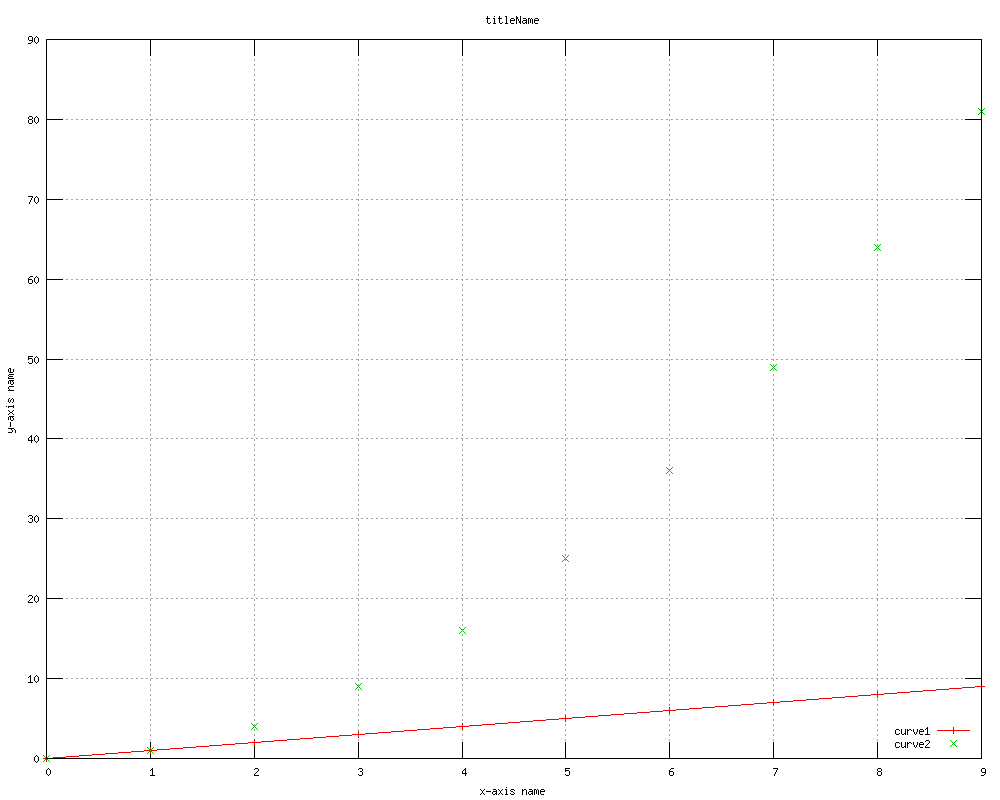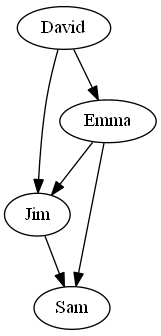An Introduction to SNAP

CS224W:
Social and Information Network Analysis
Autumn 2011

# What is SNAP?

Stanford Network Analysis Platform (SNAP) is a general purpose network analysis and graph mining library. It is written in C++ and easily scales to massive networks with hundreds of millions of nodes, and billions of edges. It efficiently manipulates large graphs, calculates structural properties, generates regular and random graphs, and supports attributes on nodes and edges. SNAP is also available through the NodeXL which is a graphical front-end that integrates network analysis into Microsoft Office and Excel.

# Installation

## SNAP in Linux

For Linux system, you have to install g++ first. Then, we have example Makefile in directory examples/kronfit. Take a look at that Makefile and you will find that under Linux, you should uncomment the two lines:

```#CXXFLAGS += -std=c++98 -Wall
#LDFLAGS += -lrt
```

Then, substitute the main program name kronfit with your own file name. Also make sure to point to the correct snap directory.

## SNAP in Windows

Under Windows system, you should install Visual Studio first. Then, open Visual Studio and create a project, add "#include YOUR_SNAP_PATH/snap/Snap.h" in your main .cpp file and include the Snap.h/cpp into your project. Also, due to the settings of SNAP, the character set must be set to Multi-byte. You can right-click on your project and go to Properties --> Configuration Properties --> General --> Projects Defaults --> Character Set --> Select "Use Multi-Byte Character Set". Then you can enjoy the powerful arsenal of SNAP!

# Data Structures in SNAP

SNAP contains a STL-like library, specified in directory glib. It contains basic data structures, like vectors, hash-tables and strings. These data structures perform efficiently and supports serialization for loading and saving.

## Basic Data Structures

• Integer: TInt
• Real number: TFlt
• String: TStr

• ```TInt a=5;
cout << a << endl; --- 5
//In C style, use printf("%d\n", a.Val);
**************************************
TStr a="abc";
TStr b="ccc";
cout << a.CStr() << endl; --- abc
cout << a.Len() << endl; --- 3
cout << a << endl;   --- a
cout << (a==b) << endl; --- 0
```

## Composite Data Structures

• Pair: TPair<Type1, Type2>
• Triple: TTriple<Type1, Type2, Type3>
• Type can also be other complex structures like TVec, TPair
• ```TPair<TInt, TFlt> a;
a.Val1=1;
a.Val2=2.3;
```

## Containers

• Vector: TVec<Type>
• Hash table: THash<Key Type, Value Type>
• Hash Set: THashSet<Key Type>
• When key is string, use TStrHash<Value Type> instead of THash<TStr, Value Type>. TStrHash uses string pool and saves more space.

• ```TVec<TInt> a;
cout << a << endl;    --- 10
cout << a.Len() << endl; --- 3
***********************************
THash<TInt, TStr> a;
cout << a.GetKey(0) << endl;         --- 12
cout << a.CStr() << endl;		 --- abc
cout << a.GetKeyId(12) << endl;		 --- 0
cout << a.GetDat(34).CStr() << endl; --- def
***********************************
THashSet<TInt> a;
cout << a.GetKey(2) << endl; --- 56
```

SNAP supports serialization for all the data structures aforementioned, as well as the network/graph structures to be introduced. It is much more efficient to store a data structure to binary files than to text format. Saving and loading binary files for data structures in SNAP is very simple, just via function Save and Load.

```TVec<TInt> a;
{TFOut fout("a.bin"); a.Save(fout);}
```

Example .cpp for basic data structure and data type in SNAP: BasicDS_DT_SNAP.cpp

# Graph Manipulation in SNAP

SNAP contains a powerful graph manupulation library, specified in directory snap. It can deal with generating, manipulating as well as analyzing graphs/networks.

## Graph/Network Types

• TUNGraph: undirected graph with no multi-edge
• TNGraph: directed graph with no multi-edge
• TNEGraph: directed graph with multi-edge
• TNodeNet<TNodeData>: directed graph with TNodeData object for each node
• TNodeEDatNet<TNodeData, TEdgeData>: directed graph with TNodeData on each node and TEdgeData on each edge
• TNodeEdgeNet<TNodeData, TEdgeData>: directed multi-edge graph with TNodeData on each node and TEdgeData on each edge
• If you use the T..Net class, make sure you implement saving and loading functions. Example: NodeNet.cpp

When creating a graph/network, use smart pointer (e.g. TPt<TNGraph> g) whenever possible to let the library automatically allocating/de-allocating the space for you. Furthermore, when adding an edge, make sure the two nodes have already been added.

```PNGraph Graph = TNGraph::New(); //PNGraph is defined in SNAP as TPt<TNGraph>
cout << Graph->GetNodes() << endl; --- 2  //number of nodes
cout << Graph->GetEdges() << endl; --- 1  //number of edges
******************************
PNGraph Graph = TSnap::GenRndGnm<PNGraph>(100, 1000);
//create a directed random graph on 100 nodes and 1,000 edges
```

## Playing with a Graph

We can use SNAP library to explore and analyze a graph easily.

```1. Traverse a graph

//traverse the nodes
for (TNGraph::TNodeI NI = Graph->BegNI(); NI < Graph->EndNI(); NI++)
printf("%d %d %d\n", NI.GetId(), NI.GetOutDeg(), NI.GetInDeg());

//traverse the edges
for (TNGraph::TEdgeI EI = Graph->BegEI(); EI < Graph->EndEI(); EI++)
printf("edge (%d, %d)\n", EI.GetSrcNId(), EI.GetDstNId());

//we can traverse the edges also like this
for (TNGraph::TNodeI NI = Graph->BegNI(); NI < Graph->EndNI(); NI++)
for (int e = 0; e < NI.GetOutDeg(); e++)
printf("edge (%d %d)\n", NI.GetId(), NI.GetOutNId(e));

2. Get properties of a graph

// generate a network using Forest Fire model
PNGraph G = TSnap::GenForestFire(1000, 0.35, 0.35);

//get the largest weakly connected component of G
PNGraph WccG = TSnap::GetMxWcc(G);

//get a subgraph induced on nodes {0,1,2,3,4}
PNGraph SubG = TSnap::GetSubGraph(G, TIntV::GetV(0,1,2,3,4));

TVec<TPair<TInt, TInt> > CntV; // vector of pairs of integers (size, count)
//get distribution of connected components (component size, count)
TSnap::GetWccSzCnt(G, CntV);

//get degree distribution pairs (degree, count)
TSnap::GetOutDegCnt(G, CntV);

//convert to undirected graph TUNGraph
PUNGraph UG = TSnap::ConvertGraph<PUNGraph, PNGraph>(G);
```

Example:
1. Generate a G(n,m) graph (Erdos-Renyi model): Gnm.cpp
2. Get size for connected components in an undirected graph: getCC.cpp

# Datasets in SNAP

SNAP contains a collection of about 50 large network datasets from tens of thousands of nodes and edges to tens of millions of nodes and edges. It includes social networks, web graphs, road networks, internet networks, citation networks, collaboration networks, and communication networks. A full list is available at http://snap.stanford.edu/data/index.html, with downloadable datasets. Some example networks include:
• Social networks: online social networks, edges represent interactions between people
• Citation networks: nodes represent papers, edges represent citations
• Collaboration networks: nodes represent scientists, edges represent collaborations (co-authoring a paper)
• Amazon networks : nodes represent products and edges link commonly co-purchased products
• Twitter and Memetracker : Memetracker phrases, links and 467 million Tweets

Loading and saving network data mentioned above is similar to that of data structures. Here's an example for data as20graph.txt in the directory example of SNAP.

```Top lines of as20graph.txt:
# Directed Node Graph
# Autonomous systems (graph is undirected, each edge is saved twice)
# Nodes: 6474 Edges: 26467
# SrcNId DstNId
1 3
1 6
1 32
1 48
1 70
1 63
...

//0 is the column id for source node
//1 is the column id for target node

Saving:
TSnap::SaveEdgeList<PUNGraph>(g, "as20graph.txt", "");
```

# Plotting and Visualization in SNAP

## Plotting in SNAP

Making a plot in SNAP requires installing the software Gnuplot. Gnuplot is a plotting software with its own grammar. Make sure that the path containing wgnuplot.exe (for Windows) or gnuplot (for Linux) is in your environmental variable \$PATH. Fortunately, SNAP contains interfaces to help you directly generate Gnuplot scripts and run Gnuplot for you. Here's a typical example of plotting two curves with known (x,y) coordinates:

```TVec<TPair<TFlt,TFlt> > XY1, XY2;
for (int i=0; i<10; ++i)
{
}
TGnuPlot Gp("fileName", "titleName");
Gp.SetXYLabel("x-axis name", "y-axis name");
Gp.SavePng(); //or Gp.SaveEps();
//You can also use log-log scale via Gp.SetScale(gpsLog10XY);
```

Here's the result:Fig. 1 Plotting line y=x and points y=x^2 using Gnuplot

Typically, the plotting interface of SNAP will generate three types of files for each plot:

• .plt file contains the plotting command for gnuplot
• .tab file contains the data
• .png or .eps is the plot
• You can manipulate .plt and .tab and then run wgnuplot.exe xx.plt (for Windows) or gnuplot xx.plt (for Linux) to replot.
Example .cpp to plot exponential and Poisson distribution using Gnuplot: Gnuplot_example.cpp

## Graph Visualization in SNAP

Visualize a graph in SNAP requires installing the software GraphViz. GraphViz is a graph-visualization software. Make sure that the path of bin in your GraphViz directory is in your environmental variable \$PATH. Like TGnuplot, SNAP contains interfaces to help you directly manipulate GraphViz. Here's a typical example of visualizing a simple directed graph:

```PNGraph g=TNGraph::New();

TIntStrH name;  //node labels